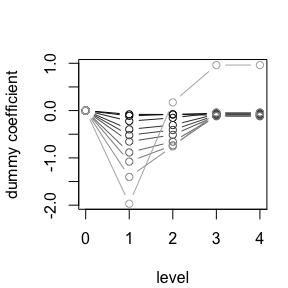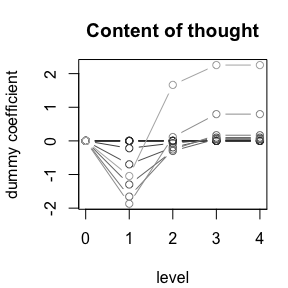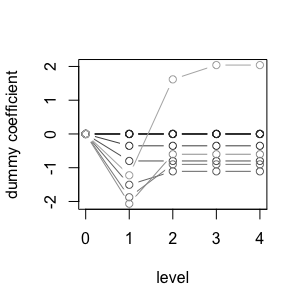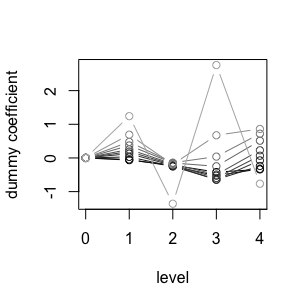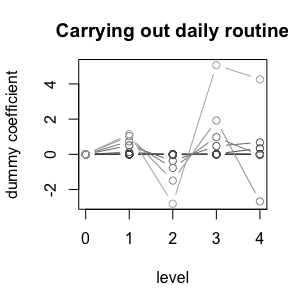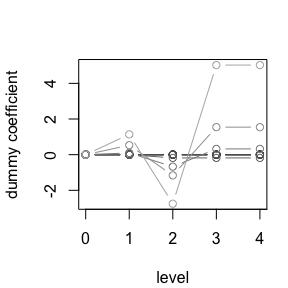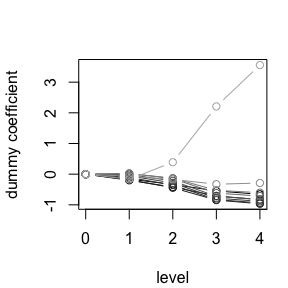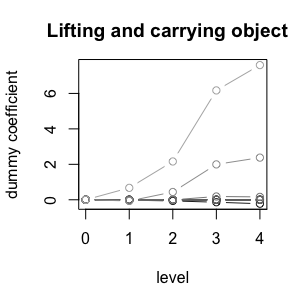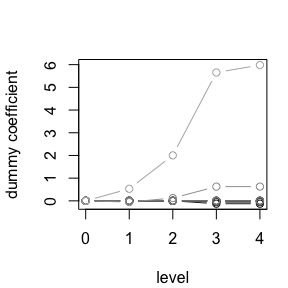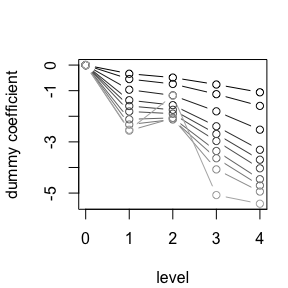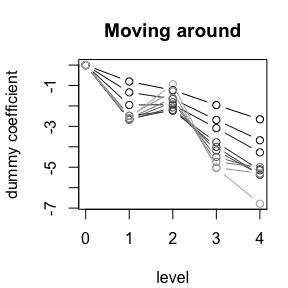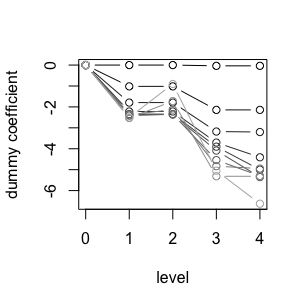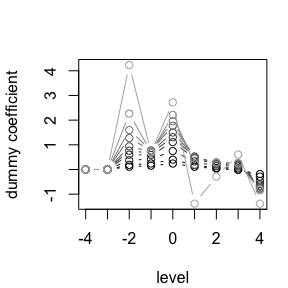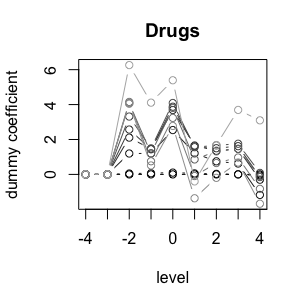# Introduction to ordPens

## Primary functions

• ordPCA
• ordFusion
• ordSelect
• ordSmooth
• ordAOV
• ordGene

The ordPens R package offers selection, and/or smoothing/fusion of ordinally scaled independent variables using a group lasso or generalized ridge penalty. In addition, nonlinear principal components analysis for ordinal variables is provided, using a second-order difference penalty.

Smoothing and selection of ordinal predictors is done by the function ordSelect(); smoothing only, by ordSmooth(); fusion and selection of ordinal predictors by ordFusion(). For ANOVA with ordinal factors, use ordAOV(). To test for genes that are differentially expressed between ordinal levels, use ordGene(). For Nonlinear PCA, performance evaluation and selection of an optimal penalty parameter, use ordPCA() and see also vignette("ordPCA", package = "ordPens").

library(ordPens) 

## Fusion, smoothing and selection

These functions fit penalized dummy coefficients of ordinally scaled independent variables. The covariates are assumed to take values 1,2,…,max, where max denotes the (columnwise) highest level observed in the data. The ordSmooth() function penalizes the sum of squared differences of adjacent dummy coefficients, while ordSelect() uses a group lasso penalty on differences of adjacent dummies and ordFusion() proceeds with a fused lasso penalty on differences of adjacent dummy coefficients using the glmpath algorithm. For details on the different penalties used, please see also Tutz and Gertheiss (2014, 2016).

ordPens extends penalization to the wider framework of generalized linear models, thus allowing for binary or Poisson distributed responses as well as the classical Gaussian model.
The response vector y can be either of numeric scale (leading to the default model = "linear"), 0/1 coded (model = "logit") or contain count data (i.e. model = "poisson").

### Smoothing

For smoothing only, the generalized linear model is fitted via penalized maximum likelihood. In particular, the logit or poisson model is fitted by penalized Fisher scoring. For stopping the iterations the criterion $$\sqrt(\sum((b.new-b.old)^2)/\sum(b.old^2)) < \delta$$ is used.

The tuning parameter $$\lambda$$ controls the overall strength of the penalty. If $$\lambda = 0$$ one obtains the usual un-penalized coefficient estimates. If $$\lambda \to \infty$$ coefficients are enforced to shrink towards 0. For $$0 < \lambda < \infty$$ parameters corresponding to adjacent categories are enforced to obtain similar values, as it is sensible to assume that coefficients vary smoothly over ordinal categories.

It should be highlighted, that ordSmooth() is intended for use with high-dimensional ordinal predictors; more precisely, if the number of ordinal predictors is large. Package ordPens, however, also includes auxiliary functions such that mgcv::gam() (Wood, 2011 and 2017) can be used for fitting generalized linear and additive models with first- and second-order ordinal smoothing penalty as well as built-in smoothing parameter selection. In addition, mgcv tools for further statistical inference can be used. Note, however, significance of smooth (ordinal) terms is only reliable in case of the second-order penalty. Also note, if using gam(), dummy coefficients/fitted functions are centered over the data observed. For details, see also Gertheiss et al. (2021).

### Selection

When many predictors are available, selecting the, possibly few, relevant ones is of particular interest. Joint selection and smoothing is achieved by a ordinal group lasso penalty, where the $$L_2$$-norm is modified by squared differences. While the ridge penalty shrinks the coefficients of correlated predictors towards each other, the (ordinal) group lasso additionally tends to select one or some of them and discard the others.
For more information on the original group lasso (for nominal predictors and grouped variables in general), cf. Meier et. al (2008).

### Fusion

If a variable is selected by the group lasso, all coefficients belonging to that selected covariate are estimated as differing (at least slightly). However, it might be useful to collapse certain categories. Clustering is done by a fused lasso type penalty using the $$L_1$$-norm on adjacent categories. That is, adjacent categories get the same coefficient values and one obtains clusters of categories. The fused lasso also enforces selection of predictors: since the parameters of the reference categories are set to zero, a predictor is excluded if all its categories are combined into one cluster.

### Simulated data example

Now, let’s demonstrate and compare the three methods for the default Gaussian model.
Simulating data with ordinal predictors:

set.seed(123)

# generate (ordinal) predictors
x1 <- sample(1:8, 100, replace = TRUE)
x2 <- sample(1:6, 100, replace = TRUE)
x3 <- sample(1:7, 100, replace = TRUE)

# the response
y <- -1 + log(x1) + sin(3*(x2-1)/pi) + rnorm(100)

# x matrix
x <- cbind(x1,x2,x3)

# lambda values
lambda <- c(1000, 500, 200, 100, 70, 50, 30, 20, 10, 1) 

The syntax of the three functions is very similar, type also ?ordSmooth or one of the other functions. The fitted ordPen object contains the fitted coefficients and can be visualized by the generic plot function:

osm1 <- ordSmooth(x = x, y = y, lambda = lambda)
osl <- ordSelect(x = x, y = y, lambda = lambda)
#> Setting update.every = length(lambda) + 1
#> Lambda: 1000  nr.var: 1
#> Lambda: 500  nr.var: 1
#> Lambda: 200  nr.var: 1
#> Lambda: 100  nr.var: 8
#> Lambda: 70  nr.var: 13
#> Lambda: 50  nr.var: 13
#> Lambda: 30  nr.var: 13
#> Lambda: 20  nr.var: 13
#> Lambda: 10  nr.var: 13
#> Lambda: 1  nr.var: 19
ofu <- ordFusion(x = x, y = y, lambda = lambda)

par(mar = c(4.1, 4.1, 3.1, 1.1))
plot(osm1)
plot(osl, main = "")
plot(ofu, main = "")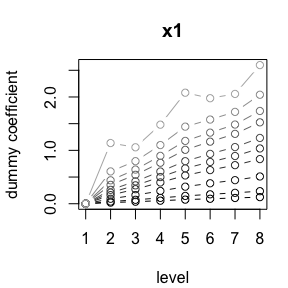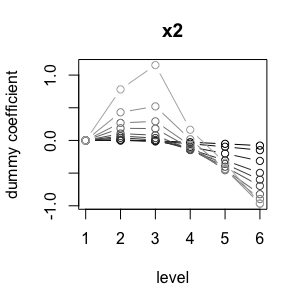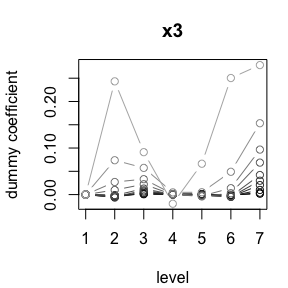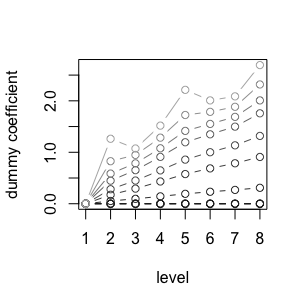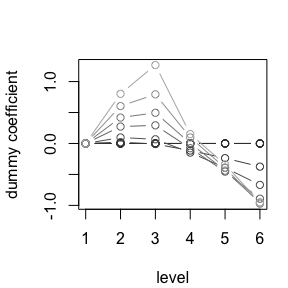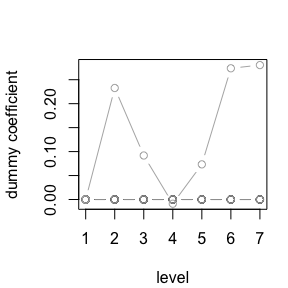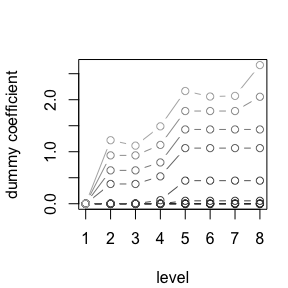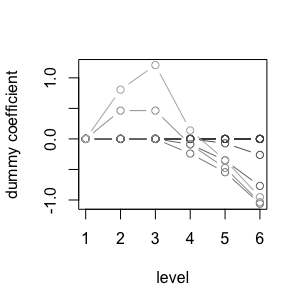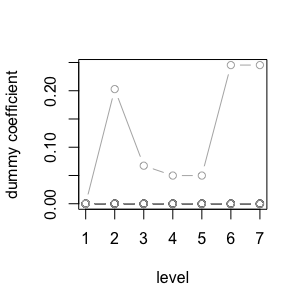The vector of penalty parameters lambda is sorted decreasingly and each curve corresponds to a $$\lambda$$ value, with larger values being associated with the darker colors. The top row corresponds to smoothing, the middle row shows selection and the bottom row demonstrates variable fusion.

It is seen that for smaller $$\lambda$$, the coefficients are more wiggly, while differences of adjacent categories are more and more shrunk when $$\lambda$$ increases, which yields smoother coefficients.

Variable 3 is excluded from the model for $$\lambda \geq 10$$ with both ordSelect() and ordFusion(). In general, ordFusion() yields estimates that tend to be flat over adjacent categories, which represents a specific form of smoothness. With the fused lasso, all categories are fused and excluded at some point, which can be viewed from the bottom row or from the matrix of estimated coefficients (not printed here):

round(osm1$coefficients, digits = 3) round(osl$coefficients, digits = 3)
round(ofu$coefficients, digits = 3) Now, let’s plot the path of coefficients against the log-lambda value: matplot(log(lambda), t(osm1$coefficients), type = "l", xlab = expression(log(lambda)),
ylab = "Coefficients", col = c(1, rep(2,8), rep(3,6), rep(4,7)), cex.main = 1,
main = "Smoothing", xlim = c(max(log(lambda)), min(log(lambda))))
axis(4, at = osm1$coefficients[,ncol(osm1$coefficients)], label = rownames(osm1$coefficients), line = -.5, las = 1, tick = FALSE, cex.axis = 0.75) matplot(log(lambda), t(osl$coefficients), type = "l", xlab = expression(log(lambda)),
ylab = "Coefficients", col = c(1, rep(2,8), rep(3,6), rep(4,7)), cex.main = 1,
main = "Selection",  xlim = c(max(log(lambda)), min(log(lambda))))
axis(4, at = osl$coefficients[,ncol(osl$coefficients)], label = rownames(osl$coefficients), line = -.5, las = 1, tick = FALSE, cex.axis = 0.75) matplot(log(lambda), t(ofu$coefficients), type = "l", xlab = expression(log(lambda)),
ylab = "Coefficients", col = c(1, rep(2,8), rep(3,6), rep(4,7)), cex.main = 1,
main = "Fusion", xlim = c(max(log(lambda)), min(log(lambda))))
axis(4, at = ofu$coefficients[,ncol(ofu$coefficients)], label = rownames(ofu$coefficients), line = -.5, las = 1, tick = FALSE, cex.axis = 0.75)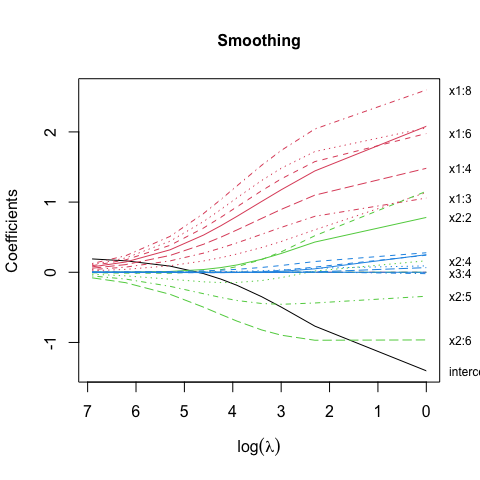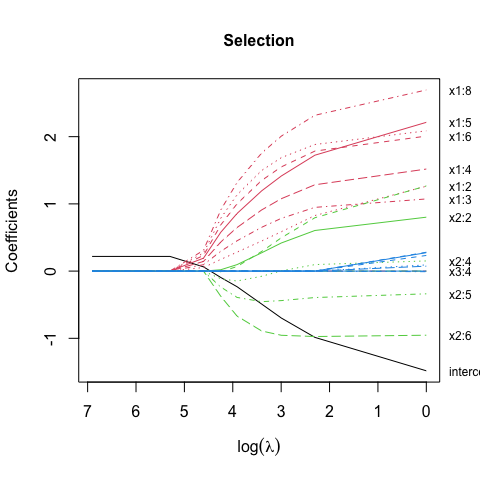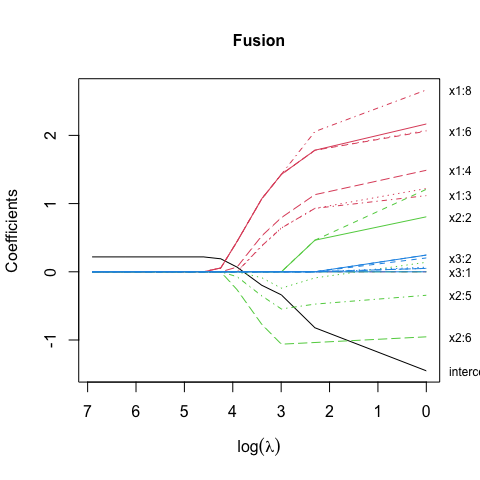Each curve corresponds to a dummy coefficient and each color corresponds to a variable (intercept not penalized here). The effect of the different penalties can be seen very clearly. #### Using mgcv::gam() with ordPens and comparison to ordSmooth() As mentioned before, the package also builds a bridge to mgcv::gam() by providing a new type of spline basis for ordered factors s(..., bs = "ordinal"), such that smooth terms in the GAM formula can be used. In addition, generic functions for prediction and plotting are provided; and existing mgcv tools for further statistical inference can be used this way, which enables a very flexible analysis. Before estimation, we need to modify the ordinal predictors to the class of ordered factors. We also add a nominal covariate to control for. x1 <- as.ordered(x1) x2 <- as.ordered(x2) x3 <- as.ordered(x3) u1 <- sample(1:8, 100, replace = TRUE) u <- cbind(u1) osm2 <- ordSmooth(x = x, y = y, u = u, lambda = lambda) Now, let us use gam() from mgcv for model fitting. Estimation with first-order penalty and smoothing parameter selection by REML: gom1 <- gam(y ~ s(x1, bs = "ordinal", m = 1) + s(x2, bs = "ordinal", m = 1) + s(x3, bs = "ordinal", m = 1) + factor(u1), method = "REML") Using second-order penalty instead: gom2 <- gam(y ~ s(x1, bs = "ordinal", m = 2) + s(x2, bs = "ordinal", m = 2) + s(x3, bs = "ordinal", m = 2) + factor(u1), method = "REML") The summary command including significance of smooth terms can be used in the usual way. Please note, the latter is only reliable for m = 2, as mentioned above. summary(gom2) #> #> Family: gaussian #> Link function: identity #> #> Formula: #> y ~ s(x1, bs = "ordinal", m = 2) + s(x2, bs = "ordinal", m = 2) + #> s(x3, bs = "ordinal", m = 2) + factor(u1) #> #> Parametric coefficients: #> Estimate Std. Error t value Pr(>|t|) #> (Intercept) -0.01402 0.37928 -0.037 0.971 #> factor(u1)2 0.23057 0.58116 0.397 0.693 #> factor(u1)3 0.54998 0.52426 1.049 0.297 #> factor(u1)4 0.31065 0.48449 0.641 0.523 #> factor(u1)5 0.15299 0.58312 0.262 0.794 #> factor(u1)6 -0.06266 0.48096 -0.130 0.897 #> factor(u1)7 0.27193 0.48767 0.558 0.579 #> factor(u1)8 0.37443 0.48599 0.770 0.443 #> #> Approximate significance of smooth terms: #> edf Ref.df F p-value #> s(x1) 1.710 2.146 15.047 2.07e-06 *** #> s(x2) 3.019 3.731 9.029 1.17e-05 *** #> s(x3) 1.000 1.000 0.100 0.753 #> --- #> Signif. codes: 0 '***' 0.001 '**' 0.01 '*' 0.05 '.' 0.1 ' ' 1 #> #> R-sq.(adj) = 0.419 Deviance explained = 49.4% #> -REML = 155.64 Scale est. = 1.3422 n = 100 We can also visualize the coefficients including confidence intervals by executing the plot function: par(mar = c(4.1, 4.1, 3.1, 1.1)) plot(osm2) plot(gom1) plot(gom2)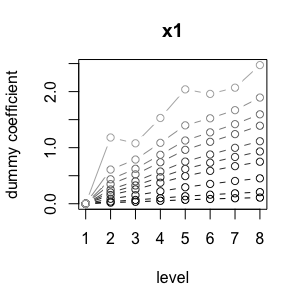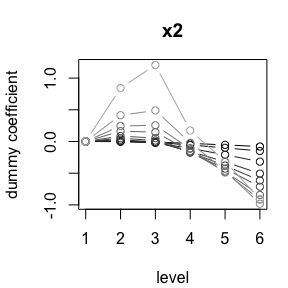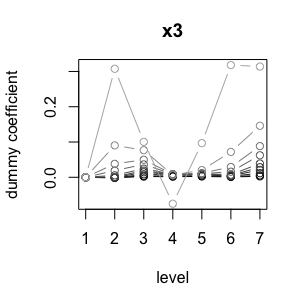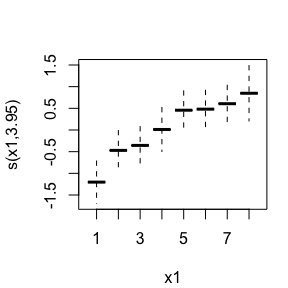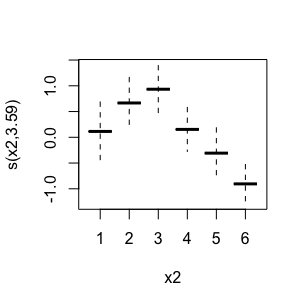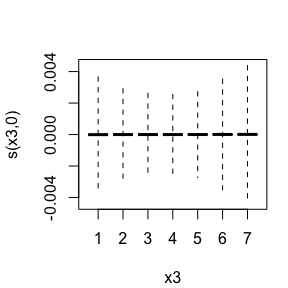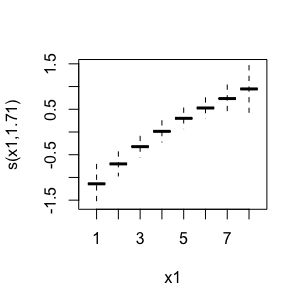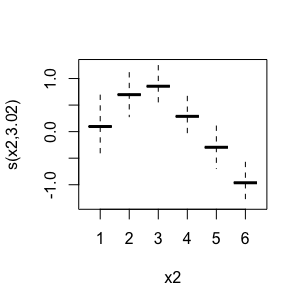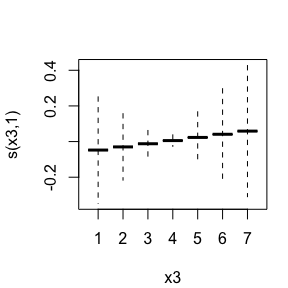Top: ordSmooth; middle row: gam with first-order penalty; bottom: gam with second-order penalty #### Predicting from an ordPen object The package also includes a generic function for prediction. It takes a fitted ordPen object and a matrix/data frame of new observations of the considered ordinal predictors (denoted by newx). If the fitted object contains also additional nominal or metric predictors, further matrices of new observations corresponding to those predictors need to be added (denoted by newu or newz). x1 <- sample(1:8, 10, replace = TRUE) x2 <- sample(1:6, 10, replace = TRUE) x3 <- sample(1:7, 10, replace = TRUE) newx <- cbind(x1, x2, x3) The type of prediction can be chosen by the type option: “link” is on the scale of linear predictors, “response” is on the scale of the response variable (inverse link function). Predict from an ordPen object: round(predict(osm1, newx), digits = 3) #> 1000 500 200 100 70 50 30 20 10 1 #> [1,] 0.261 0.301 0.404 0.539 0.632 0.733 0.911 1.068 1.351 2.107 #> [2,] 0.229 0.239 0.265 0.299 0.323 0.351 0.400 0.445 0.518 0.525 #> [3,] 0.289 0.352 0.511 0.706 0.828 0.954 1.151 1.305 1.547 2.049 #> [4,] 0.240 0.261 0.315 0.388 0.440 0.497 0.601 0.693 0.857 1.210 #> [5,] 0.211 0.203 0.178 0.137 0.106 0.070 0.007 -0.045 -0.113 -0.017 #> [6,] 0.232 0.244 0.273 0.306 0.325 0.343 0.365 0.375 0.377 0.297 #> [7,] 0.234 0.247 0.280 0.315 0.333 0.348 0.364 0.371 0.379 0.472 #> [8,] 0.272 0.320 0.441 0.588 0.680 0.772 0.910 1.007 1.124 1.094 #> [9,] 0.256 0.290 0.376 0.482 0.548 0.615 0.718 0.792 0.884 0.827 #> [10,] 0.246 0.272 0.333 0.405 0.447 0.486 0.537 0.563 0.572 0.402 round(predict(osl, newx), digits = 3) #> 1000 500 200 100 70 50 30 20 10 1 #> [1,] 0.218 0.218 0.218 0.255 0.473 0.692 0.996 1.212 1.532 2.276 #> [2,] 0.218 0.218 0.218 0.159 0.220 0.315 0.434 0.505 0.566 0.483 #> [3,] 0.218 0.218 0.218 0.331 0.683 0.970 1.299 1.485 1.691 2.102 #> [4,] 0.218 0.218 0.218 0.206 0.329 0.483 0.712 0.875 1.092 1.292 #> [5,] 0.218 0.218 0.218 0.117 0.079 0.050 -0.043 -0.113 -0.160 0.053 #> [6,] 0.218 0.218 0.218 0.296 0.356 0.378 0.403 0.413 0.406 0.260 #> [7,] 0.218 0.218 0.218 0.372 0.440 0.416 0.375 0.355 0.359 0.490 #> [8,] 0.218 0.218 0.218 0.331 0.578 0.755 0.930 0.994 0.994 1.034 #> [9,] 0.218 0.218 0.218 0.296 0.478 0.622 0.781 0.856 0.896 0.767 #> [10,] 0.218 0.218 0.218 0.331 0.455 0.511 0.552 0.551 0.504 0.356 round(predict(ofu, newx), digits = 3) #> 1000 500 200 100 70 50 30 20 10 1 #> [1,] 0.218 0.218 0.218 0.218 0.246 0.512 0.872 1.090 1.425 2.169 #> [2,] 0.218 0.218 0.218 0.218 0.190 0.069 0.183 0.303 0.572 0.538 #> [3,] 0.218 0.218 0.218 0.218 0.246 0.512 0.872 1.090 1.425 2.032 #> [4,] 0.218 0.218 0.218 0.218 0.190 0.137 0.329 0.455 0.774 1.295 #> [5,] 0.218 0.218 0.218 0.218 0.190 0.069 0.183 0.303 0.109 0.014 #> [6,] 0.218 0.218 0.218 0.218 0.246 0.440 0.519 0.545 0.488 0.315 #> [7,] 0.218 0.218 0.218 0.218 0.246 0.250 0.102 0.028 0.199 0.463 #> [8,] 0.218 0.218 0.218 0.218 0.246 0.512 0.785 0.850 0.872 1.005 #> [9,] 0.218 0.218 0.218 0.218 0.246 0.512 0.785 0.850 0.872 0.816 #> [10,] 0.218 0.218 0.218 0.218 0.246 0.440 0.519 0.545 0.488 0.344 #### ICF core set for chronic widespread Pain Lastly, we demonstrate the three methods on a publicly available dataset suiting the high-dimensional setting they’re designed for. The ICF core set for chronic widespread pain (CWP) consists of 67 rating scales - called ICF categories - and a physical health component summary measure (phcs). Each ICF factor is associated with one of the following four types: ‘body functions’, ‘body structures’, ‘activities and participation’, and ‘environmental factors’. The latter are measured on a nine-point Likert scale ranging from −4 ‘complete barrier’ to +4 ‘complete facilitator’. All remaining factors are evaluated on a five-point Likert scale ranging from 0 ‘no problem’ to 4 ‘complete problem’. Type ?ICFCoreSetCWP and see also references therein for further details. data(ICFCoreSetCWP) head(ICFCoreSetCWP) #> b1602 b122 b126 b130 b134 b140 b147 b152 b164 b180 b260 b265 b270 b280 b430 #> 1 0 1 2 1 1 0 0 2 0 0 0 0 0 1 0 #> 2 3 2 2 3 3 2 3 3 3 1 2 2 2 2 0 #> 3 0 1 2 1 1 0 1 2 0 0 0 0 0 1 0 #> 4 0 0 0 2 1 0 0 0 0 0 0 0 0 3 0 #> 5 0 0 0 0 0 0 0 0 0 0 0 0 0 0 0 #> 7 0 1 0 2 3 3 2 3 2 2 0 0 3 3 0 #> b455 b640 b710 b730 b735 b740 b760 b780 d160 d175 d220 d230 d240 d410 d415 #> 1 1 0 0 1 0 0 0 1 0 0 1 0 1 0 0 #> 2 3 0 2 3 3 3 3 3 3 2 3 3 3 3 3 #> 3 1 0 0 1 0 1 0 1 0 0 0 1 1 0 0 #> 4 3 0 0 0 0 3 0 1 0 0 0 1 0 0 2 #> 5 0 1 0 0 0 0 0 0 0 0 0 0 0 0 0 #> 7 3 2 0 3 3 3 0 2 2 2 3 2 0 3 3 #> d430 d450 d455 d470 d475 d510 d540 d570 d620 d640 d650 d660 d720 d760 d770 #> 1 1 0 2 0 1 0 0 0 1 0 0 0 0 0 0 #> 2 3 3 3 3 0 2 2 3 3 3 3 3 3 3 0 #> 3 1 0 2 0 1 0 0 0 0 0 0 0 0 0 0 #> 4 2 3 2 0 0 0 0 0 0 1 0 0 0 0 0 #> 5 0 0 0 0 0 0 0 0 0 0 0 0 0 0 0 #> 7 3 1 3 1 3 1 1 1 1 3 2 0 1 0 3 #> d845 d850 d855 d910 d920 e1101 e310 e325 e355 e410 e420 e425 e430 e450 e455 #> 1 0 0 0 0 1 0 0 0 4 0 0 0 0 4 0 #> 2 0 0 2 2 2 2 3 2 3 3 3 3 3 3 3 #> 3 0 0 0 0 1 0 0 0 4 0 0 0 0 4 0 #> 4 0 0 0 0 0 0 2 1 2 0 -1 -1 0 2 2 #> 5 0 0 0 0 0 0 1 0 1 0 0 0 0 1 0 #> 7 2 2 0 0 2 3 3 3 3 3 2 1 -1 2 2 #> e460 e465 e570 e575 e580 e590 s770 phcs #> 1 0 0 3 3 4 3 0 44.33 #> 2 2 2 2 2 2 2 2 21.09 #> 3 0 0 3 3 4 0 0 41.74 #> 4 -1 0 0 2 2 1 1 33.96 #> 5 0 0 0 0 0 0 0 46.29 #> 7 -2 -2 1 1 1 1 1 29.89 Before estimation, we make sure that the ordinal design matrix is coded adequately: y <- ICFCoreSetCWP$phcs
x <- ICFCoreSetCWP[, 1:67] + matrix(c(rep(1, 50), rep(5, 16), 1),
nrow(ICFCoreSetCWP), 67,
byrow = TRUE)
xnames <- names(x)
#>   b1602 b122 b126 b130 b134 b140 b147 b152 b164 b180 b260 b265 b270 b280 b430
#> 1     1    2    3    2    2    1    1    3    1    1    1    1    1    2    1
#> 2     4    3    3    4    4    3    4    4    4    2    3    3    3    3    1
#> 3     1    2    3    2    2    1    2    3    1    1    1    1    1    2    1
#> 4     1    1    1    3    2    1    1    1    1    1    1    1    1    4    1
#> 5     1    1    1    1    1    1    1    1    1    1    1    1    1    1    1
#> 7     1    2    1    3    4    4    3    4    3    3    1    1    4    4    1
#>   b455 b640 b710 b730 b735 b740 b760 b780 d160 d175 d220 d230 d240 d410 d415
#> 1    2    1    1    2    1    1    1    2    1    1    2    1    2    1    1
#> 2    4    1    3    4    4    4    4    4    4    3    4    4    4    4    4
#> 3    2    1    1    2    1    2    1    2    1    1    1    2    2    1    1
#> 4    4    1    1    1    1    4    1    2    1    1    1    2    1    1    3
#> 5    1    2    1    1    1    1    1    1    1    1    1    1    1    1    1
#> 7    4    3    1    4    4    4    1    3    3    3    4    3    1    4    4
#>   d430 d450 d455 d470 d475 d510 d540 d570 d620 d640 d650 d660 d720 d760 d770
#> 1    2    1    3    1    2    1    1    1    2    1    1    1    1    1    1
#> 2    4    4    4    4    1    3    3    4    4    4    4    4    4    4    1
#> 3    2    1    3    1    2    1    1    1    1    1    1    1    1    1    1
#> 4    3    4    3    1    1    1    1    1    1    2    1    1    1    1    1
#> 5    1    1    1    1    1    1    1    1    1    1    1    1    1    1    1
#> 7    4    2    4    2    4    2    2    2    2    4    3    1    2    1    4
#>   d845 d850 d855 d910 d920 e1101 e310 e325 e355 e410 e420 e425 e430 e450 e455
#> 1    1    1    1    1    2     5    5    5    9    5    5    5    5    9    5
#> 2    1    1    3    3    3     7    8    7    8    8    8    8    8    8    8
#> 3    1    1    1    1    2     5    5    5    9    5    5    5    5    9    5
#> 4    1    1    1    1    1     5    7    6    7    5    4    4    5    7    7
#> 5    1    1    1    1    1     5    6    5    6    5    5    5    5    6    5
#> 7    3    3    1    1    3     8    8    8    8    8    7    6    4    7    7
#>   e460 e465 e570 e575 e580 e590 s770
#> 1    5    5    8    8    9    8    1
#> 2    7    7    7    7    7    7    3
#> 3    5    5    8    8    9    5    1
#> 4    4    5    5    7    7    6    2
#> 5    5    5    5    5    5    5    1
#> 7    3    3    6    6    6    6    2

We can check whether all categories are observed at least once as follows:

rbind(apply(x, 2, min), apply(x, 2, max))
#>      b1602 b122 b126 b130 b134 b140 b147 b152 b164 b180 b260 b265 b270 b280
#> [1,]     1    1    1    1    1    1    1    1    1    1    1    1    1    1
#> [2,]     4    5    4    5    5    5    5    5    5    4    4    5    5    5
#>      b430 b455 b640 b710 b730 b735 b740 b760 b780 d160 d175 d220 d230 d240 d410
#> [1,]    1    1    1    1    1    1    1    1    1    1    1    1    1    1    1
#> [2,]    4    5    5    5    5    5    5    4    5    4    5    5    5    5    5
#>      d415 d430 d450 d455 d470 d475 d510 d540 d570 d620 d640 d650 d660 d720 d760
#> [1,]    1    1    1    1    1    1    1    1    1    1    1    1    1    1    1
#> [2,]    4    5    4    5    5    5    4    4    4    5    5    5    5    4    5
#>      d770 d845 d850 d855 d910 d920 e1101 e310 e325 e355 e410 e420 e425 e430
#> [1,]    1    1    1    1    1    1     2    1    1    2    1    1    1    1
#> [2,]    5    5    5    5    5    5     9    9    9    9    9    9    9    9
#>      e450 e455 e460 e465 e570 e575 e580 e590 s770
#> [1,]    1    2    1    1    1    1    1    1    1
#> [2,]    9    9    9    9    9    9    9    9    5

As for some covariates not all possible levels are observed at least once, the easiest way is to add a corresponding row to x with corresponding y value being NA to ensure a corresponding coefficient is to be fitted.

x <- rbind(x, rep(1,67))
x <- rbind(x, c(rep(5, 50), rep(9,16), 5))
y <- c(y, NA, NA)

osm_icf <- ordSmooth(x = x, y = y, lambda = lambda)
osl_icf <- ordSelect(x = x, y = y, lambda = lambda)
#> Setting update.every = length(lambda) + 1
#> Lambda: 1000  nr.var: 20
#> Lambda: 500  nr.var: 55
#> Lambda: 200  nr.var: 160
#> Lambda: 100  nr.var: 268
#> Lambda: 70  nr.var: 279
#> Lambda: 50  nr.var: 302
#> Lambda: 30  nr.var: 305
#> Lambda: 20  nr.var: 313
#> Lambda: 10  nr.var: 317
#> Lambda: 1  nr.var: 317
ofu_icf <- ordFusion(x = x, y = y, lambda = lambda) 

Let us illustrate the fitted coefficients for some covariates for different values of $$\lambda$$ (larger values being associated with the darker curves). We can use axis() to replace the integers 1,2,… used for fitting with the original ICF levels:

wx <- which(xnames=="b1602"|xnames=="d230"|xnames=="d430"|xnames=="d455"|xnames=="e1101")
xmain <- c()
xmain[wx] <- c("Content of thought",
"Carrying out daily routine",
"Lifting and carrying objects",
"Moving around",
"Drugs")

par(mar = c(4.1, 4.1, 3.1, 1.1))
for(i in wx){
plot(osm_icf, whx = i, main = "", xaxt = "n")
axis(1, at = 1:length(osm_icf$xlevels), labels = ((1:length(osm_icf$xlevels)) - c(rep(1,50), rep(5,16), 1)[i]))

plot(osl_icf, whx = i, main = xmain[i], xaxt = "n")
axis(1, at = 1:length(osm_icf$xlevels), labels = ((1:length(osm_icf$xlevels)) - c(rep(1,50), rep(5,16), 1)[i]))

plot(ofu_icf, whx = i, main = "", xaxt = "n")
axis(1, at = 1:length(osm_icf$xlevels), labels = ((1:length(osm_icf$xlevels)) - c(rep(1,50), rep(5,16), 1)[i]))
}